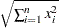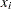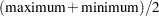The STDIZE Procedure
 Computation of the Statistics

Formulas for statistics of METHOD=MEAN, METHOD=MEDIAN, METHOD=SUM, METHOD=USTD, METHOD=STD, METHOD=RANGE, and METHOD=IQR are given in the chapter "Elementary Statistics Procedures" (Base SAS Procedures Guide).

Note that the computations of median and upper and lower quartiles depend on the PCTLMTD= option.

The other statistics listed in Table 82.2, except for METHOD=IN, are described as follows:

EUCLEN

Euclidean length., whereis theth observation andis the total number of observations in the sample.

L()

Minkowski metric. This metric is documented as the LEAST=option in the PROC FASTCLUS statement of the FASTCLUS procedure (see Chapter 34, The FASTCLUS Procedure ).

If you specify METHOD=L() in the PROC STDIZE statement, your results are similar to those obtained from PROC FASTCLUS if you specify the LEAST=p option with MAXCLUS=1 (and use the default values of the MAXITER= option). The difference between the two types of calculations concerns the maximum number of iterations. In PROC STDIZE, it is a criterion for convergence on all variables; in PROC FASTCLUS, it is a criterion for convergence on a single variable.

The location and scale measures for L() are output to the OUTSEED= data set in PROC FASTCLUS.

MIDRANGEABW()

Tukey’s biweight. Refer to Goodall (1983, pp. 376–378, p. 385) for the biweight 1-step M-estimate. Also refer to Iglewicz (1983, pp. 416-418) for the biweight A-estimate.

AHUBER()

Hubers. Refer to Goodall (1983, pp. 371–374) for the Huber 1-step M-estimate. Also refer to Iglewicz (1983, pp. 416-418) for the Huber A-estimate of scale.

AWAVE()

Andrews’ wave. Refer to Goodall (1983, p. 376) for the Wave 1-step M-estimate. Also refer to Iglewicz (1983, pp. 416-418) for the Wave A-estimate of scale.

AGK()

The noniterative univariate form of the estimator described by Art, Gnanadesikan, and Kettenring (1982).

The AGK estimate is documented in the section on the METHOD= option in the PROC ACECLUS statement of the ACECLUS procedure (also see the section Background in Chapter 22, The ACECLUS Procedure ). Specifying METHOD=AGK() in the PROC STDIZE statement is the same as specifying METHOD=COUNT and P=in the PROC ACECLUS statement.

SPACING()

The absolute difference between two data values. The minimum spacing for a proportionis the minimum absolute difference between two data values that contain a proportionof the data between them. The mid-minimum spacing is the mean of these two data values.Previous Page | Next Page | Top of Page